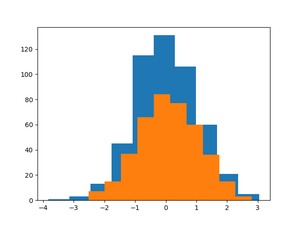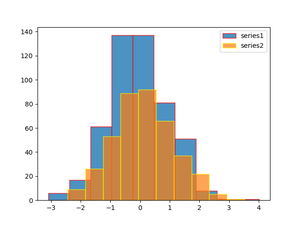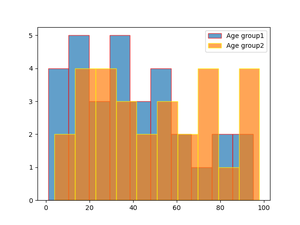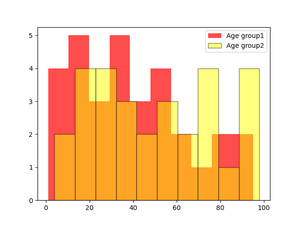Related Articles
How to plot two histograms together in Matplotlib?
• Difficulty Level : Expert
• Last Updated : 11 Dec, 2020

Prerequisites:

The histogram is the graphical representation that organizes grouped data points into the specified range. Creating the histogram provides the visual representation of data distribution. By using a histogram we can represent the large amount of data and it’s frequency as one continuous plot.

### Function used

For creating the Histogram in Matplotlib we use hist() function which belongs to pyplot module. For plotting two histograms together, we have to use hist() function separately with two datasets by giving some setting.

Syntax:

matplotlib.pyplot.hist(x, bins, edgecolor color, label)

Parameter:

### Approach

• Import module
• Create or load data for two datasets
• Plot histogram with both dataframes separately
• Plot them together

Example 1:

## Python

 `# importing libraries``import` `matplotlib.pyplot as plt``import` `numpy as np`` ` `# generating two series of random ``# values using numpy random module ``# of shape (500,1)``series1 ``=` `np.random.randn(``500``, ``1``)``series2 ``=` `np.random.randn(``400``, ``1``)`` ` `# plotting first histogram``plt.hist(series1)`` ` `# plotting second histogram``plt.hist(series2)`` ` `# Showing the plot using plt.show()``plt.show()`

Output:Example 2:

## Python

 `# importing libraries``import` `matplotlib.pyplot as plt``import` `numpy as np``from` `numpy.lib.histograms ``import` `histogram`` ` `# generating two series of random values ``# using numpy random module of shape (500,1)``series1 ``=` `np.random.randn(``500``, ``1``)``series2 ``=` `np.random.randn(``400``, ``1``)`` ` `# plotting first histogram``plt.hist(series1, label``=``'series1'``, alpha``=``.``8``, edgecolor``=``'red'``)`` ` `# plotting second histogram``plt.hist(series2, label``=``'series2'``, alpha``=``0.7``, edgecolor``=``'yellow'``)``plt.legend()`` ` `# Showing the plot using plt.show()``plt.show()`

Output:Example 3: Histograms representing two age groups

## Python

 `# importing libraries``import` `matplotlib.pyplot as plt`` ` `# giving two age groups data``age_g1 ``=` `[``1``, ``3``, ``5``, ``10``, ``15``, ``17``, ``18``, ``16``, ``19``, ``21``,``          ``23``, ``28``, ``30``, ``31``, ``33``, ``38``, ``32``, ``40``, ``45``, ``          ``43``, ``49``, ``55``, ``53``, ``63``, ``66``, ``85``, ``80``, ``57``, ``          ``75``, ``93``, ``95``]`` ` `age_g2 ``=` `[``6``, ``4``, ``15``, ``17``, ``19``, ``21``, ``28``, ``23``, ``31``, ``36``,``          ``39``, ``32``, ``50``, ``56``, ``59``, ``74``, ``79``, ``34``, ``98``, ``97``,``          ``95``, ``67``, ``69``, ``92``, ``45``, ``55``, ``77``, ``76``, ``85``]`` ` `# plotting first histogram``plt.hist(age_g1, label``=``'Age group1'``, alpha``=``.``7``, edgecolor``=``'red'``)`` ` `# plotting second histogram``plt.hist(age_g2, label``=``'Age group2'``, alpha``=``0.7``, edgecolor``=``'yellow'``)``plt.legend()`` ` `# Showing the plot using plt.show()``plt.show()`

Output:Example 4:

## Python

 `# importing libraries``import` `matplotlib.pyplot as plt`` ` `# giving two age groups data``age_g1 ``=` `[``1``, ``3``, ``5``, ``10``, ``15``, ``17``, ``18``, ``16``, ``19``,``          ``21``, ``23``, ``28``, ``30``, ``31``, ``33``, ``38``, ``32``, ``          ``40``, ``45``, ``43``, ``49``, ``55``, ``53``, ``63``, ``66``, ``          ``85``, ``80``, ``57``, ``75``, ``93``, ``95``]`` ` `age_g2 ``=` `[``6``, ``4``, ``15``, ``17``, ``19``, ``21``, ``28``, ``23``, ``31``, ``          ``36``, ``39``, ``32``, ``50``, ``56``, ``59``, ``74``, ``79``, ``34``, ``          ``98``, ``97``, ``95``, ``67``, ``69``, ``92``, ``45``, ``55``, ``77``,``          ``76``, ``85``]`` ` `# plotting first histogram``plt.hist(age_g1, label``=``'Age group1'``, bins``=``14``, alpha``=``.``7``, edgecolor``=``'red'``)`` ` `# plotting second histogram``plt.hist(age_g2, label``=``"Age group2"``, bins``=``14``, alpha``=``.``7``, edgecolor``=``'yellow'``)``plt.legend()`` ` `# Showing the plot using plt.show()``plt.show()`

Output:Example 5: Changing bar color from default

## Python

 `# importing libraries``import` `matplotlib.pyplot as plt`` ` `# giving two age groups data``age_g1 ``=` `[``1``, ``3``, ``5``, ``10``, ``15``, ``17``, ``18``, ``16``, ``19``, ``21``,``          ``23``, ``28``, ``30``, ``31``, ``33``, ``38``, ``32``, ``40``, ``45``, ``          ``43``, ``49``, ``55``, ``53``, ``63``, ``66``, ``85``, ``80``, ``57``, ``          ``75``, ``93``, ``95``]`` ` `age_g2 ``=` `[``6``, ``4``, ``15``, ``17``, ``19``, ``21``, ``28``, ``23``, ``31``, ``36``,``          ``39``, ``32``, ``50``, ``56``, ``59``, ``74``, ``79``, ``34``, ``98``, ``97``,``          ``95``, ``67``, ``69``, ``92``, ``45``, ``55``, ``77``, ``76``, ``85``]`` ` `# plotting first histogram``plt.hist(age_g1, label``=``'Age group1'``, alpha``=``.``7``, color``=``'red'``)`` ` `# plotting second histogram``plt.hist(age_g2, label``=``"Age group2"``, alpha``=``.``5``,``         ``edgecolor``=``'black'``, color``=``'yellow'``)``plt.legend()`` ` `# Showing the plot using plt.show()``plt.show()`

Output:Attention geek! Strengthen your foundations with the Python Programming Foundation Course and learn the basics.

To begin with, your interview preparations Enhance your Data Structures concepts with the Python DS Course.

My Personal Notes arrow_drop_up